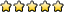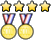## Hovercraft B/C

UTF-8 U+6211 U+662F
Exalted MemberPosts: 1518
Joined: January 18th, 2015, 7:42 am
Division: C
State: PA

### Re: Hovercraft B/C

If nobody posts a question in this long, you can.
Derive a formula for the acceleration of the blocks in a real atwood machine with two masses of mass $m1$ and $m2$and a pulley in the shape of a uniform disk of mass $M$ and radius $r$. The string is massless. Ignore friction.
The acceleration of m1 where positive is down is
EDIT: Whoops, didn't see other answer

PW240.3
MemberPosts: 1
Joined: May 7th, 2018, 2:14 pm
Division: B
State: TX

### Re: Hovercraft B/C

If nobody posts a question in this long, you can.
Derive a formula for the acceleration of the blocks in a real atwood machine with two masses of mass $m1$ and $m2$and a pulley in the shape of a uniform disk of mass $M$ and radius $r$. The string is massless. Ignore friction.

Since you have to account for the pulley's moment of inertia, wouldn't the a be a=(m1-m2)g/(m1+m2+M/4)

IvanGe
MemberPosts: 196
Joined: September 29th, 2016, 1:56 pm
Division: B
State: NY
Location: Ward Melville

### Re: Hovercraft B/C

I'm just going to ask a question because no one's verified the answer.

With what constant velocity can a 4.00 hp motor raise a mass of 125 kg?
gelinas 2016-2019, wmhs'22

nats '19:
5th - potions
5th - fossils
9th - buggy

ModeratorPosts: 477
Joined: December 6th, 2013, 1:56 pm
State: TX
Location: Austin, Texas

### Re: Hovercraft B/C

I'm just going to ask a question because no one's verified the answer.

With what constant velocity can a 4.00 hp motor raise a mass of 125 kg?
2.43 meters per second
University of Texas at Austin '22
Seven Lakes High School '18
Beckendorff Junior High '14

IvanGe
MemberPosts: 196
Joined: September 29th, 2016, 1:56 pm
Division: B
State: NY
Location: Ward Melville

### Re: Hovercraft B/C

I'm just going to ask a question because no one's verified the answer.

With what constant velocity can a 4.00 hp motor raise a mass of 125 kg?
2.43 meters per second

that's what i got, but the answer key said 2.76 m/s?

gelinas 2016-2019, wmhs'22

nats '19:
5th - potions
5th - fossils
9th - buggy

UTF-8 U+6211 U+662F
Exalted MemberPosts: 1518
Joined: January 18th, 2015, 7:42 am
Division: C
State: PA

### Re: Hovercraft B/C

I'm just going to ask a question because no one's verified the answer.

With what constant velocity can a 4.00 hp motor raise a mass of 125 kg?
2.43 meters per second
Can you explain this one

Tesel
Exalted MemberPosts: 161
Joined: January 30th, 2016, 8:03 pm
Division: C
State: MI

### Re: Hovercraft B/C

I'm just going to ask a question because no one's verified the answer.

With what constant velocity can a 4.00 hp motor raise a mass of 125 kg?
2.43 meters per second
Can you explain this one
So the first thing to understand is that hp is a unit of power, just not an SI unit. 1 hp = 745.7 W.
From here, we can use this relationship P = Fv to solve the equation.

The reason this works is that power is really a unit of energy per time. Energy, meanwhile, is a unit of force over a distance. So, power is a unit of force over a distance per time; the distance per time corresponds to velocity.

2018 MI Mission Possible State Champions

UTF-8 U+6211 U+662F
Exalted MemberPosts: 1518
Joined: January 18th, 2015, 7:42 am
Division: C
State: PA

### Re: Hovercraft B/C

2.43 meters per second
Can you explain this one
So the first thing to understand is that hp is a unit of power, just not an SI unit. 1 hp = 745.7 W.
From here, we can use this relationship P = Fv to solve the equation.

The reason this works is that power is really a unit of energy per time. Energy, meanwhile, is a unit of force over a distance. So, power is a unit of force over a distance per time; the distance per time corresponds to velocity.
Ah, I see. Thanks!

ModeratorPosts: 477
Joined: December 6th, 2013, 1:56 pm
State: TX
Location: Austin, Texas

### Re: Hovercraft B/C

I'm just going to ask a question because no one's verified the answer.

With what constant velocity can a 4.00 hp motor raise a mass of 125 kg?
2.43 meters per second

that's what i got, but the answer key said 2.76 m/s?

Suppose I have a bowling ball with a diameter of 25 centimeters. What is the largest mass it can have such that it floats in corn syrup (specific gravity = 1.4)?
University of Texas at Austin '22
Seven Lakes High School '18
Beckendorff Junior High '14

UTF-8 U+6211 U+662F
Exalted MemberPosts: 1518
Joined: January 18th, 2015, 7:42 am
Division: C
State: PA

### Re: Hovercraft B/C

Suppose I have a bowling ball with a diameter of 25 centimeters. What is the largest mass it can have such that it floats in corn syrup (specific gravity = 1.4)?


Last edited by UTF-8 U+6211 U+662F on May 10th, 2018, 7:11 pm, edited 1 time in total.